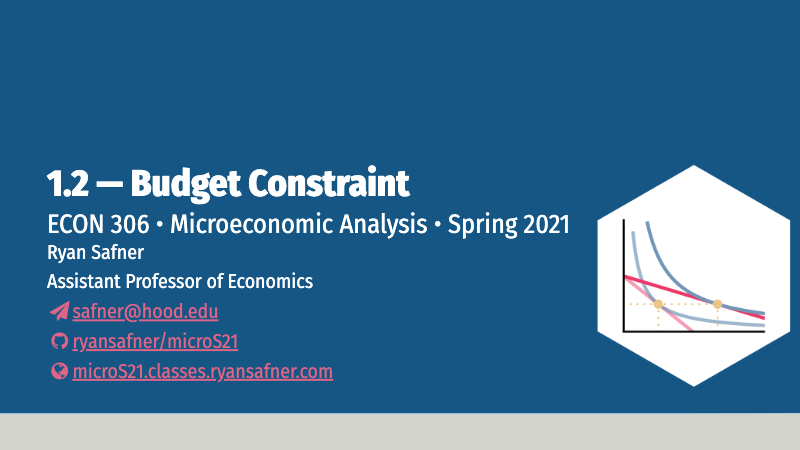1.2 — Budget Constraint — Class Content

Contents

• Section 1: Wednesday, February 3, 2021
• Section 2: Thursday, February 4, 2021

Overview

We are diving right into what is commonly called “Consumer Theory” in textbooks. While our paradigmatic example will indeed be a consumer deciding what goods and services to purchase, please recognize that this model can be applied to just about any decision made by an individual. We should more appropriately describe it as “Rational Choice Theory”. This will take several classes to fully unpack as our formal model of constrained optimization, so today we are only focusing on the constraints using the tool known as the “budget constraint.”

While budget constraints are fairly straightforward, consumer theory often the most “alien” material to students of economics: preferences, indifference curves, and utility functions derived from behavioral axioms. The model we put together—constrained optimization—is borrowed from calculus and engineering. In my opinion, a good chunk of consumer theory, particularly about preferences (next class), is a waste of your time and will give you unnecessary anxiety. It’s perhaps neat for you to see how economists ground all of their models in utility-maximization, but our time is better spent on more productive things. If, however, you plan on going to graduate school for economics or social science, read this chapter, and my online appendices, in great detail.And see this tweet for an 100% accurate example of what you can expect in a common Ph.D textbook discussing preferences.

As it is still only the first week of class: if you have not done so, please watch my welcome video, read the announcements in Blackboard, take my survey, and join our class slack channel.

Now that we are picking up the intensity and working with some models (many will be new to you at first), I recommend looking at the textbook readings before each class.

If you are following the textbook, we are going a bit out of order in two ways. It ggoes straight into (a review of principles, really) supply & demand in Chapters 1-3. We will ultimately get to supply and demand, but if you trust me, by the time we do later this semester, you will have a much richer understanding and appreciation for them, having understood how they represent the decisions by optimizing individuals as buyers and sellers.

It begins “Consumer Theory” as chapter 4. It also stars with the “newer” and “weirder” stuff first — indifference curves and utility functions, and then teaches budget constraints second. I will go in the opposite order to slowly acclimate you from familiar territory (linear equations) into newer tools and concepts (nonlinear functions, multivariate functions, optimization, etc). This is also a good time to review my Math Review Guide for next week.

The Bastiat reading is perhaps one of the greatest economic parables ever written, and demonstrates the importance of opportunity cost in clear thinking (especially about policy).

SlidesPractice

Today we will be working on practice problems. Answers will be posted later on that page.

Assignments

Preliminary Survey

Again, please take the preliminary survey on your math background by 11:59 PM Sunday. This will help us all have a productive course together.

Math Appendix

Budget Constraint for $$n$$ Goods

While we can derive a lot of useful propositions and testable claims using a simple model with just 2 goods, obviously there are many orders of magnitude more goods in an economy. Economics at the graduate and professional level begins with abstract models of $$n$$ number of goods in an economy. Naturally, we cannot graph this, so we must deal in abstract equations and set theory:

Let $$\{x_1, x_2, \cdots, x_n\}$$ denote the set of $$n$$ goods in an economy. Let $$\{p_1,p_2, \cdots, p_n\}$$ denote the set of market prices affiliated with each good. Let $$m$$ again denote an individual’s income.

For $$n$$ goods, the budget set is defined as:

$p_1x_1 + p_2x_2 + \cdots + p_n x_n \leq m$

Which we can simplify, using summation notation, as:

$\sum^{n}_{i=1}p_ix_i \leq m$

To get the limit of this set, the budget constraint, make it an equality:

$\sum^{n}_{i=1}p_ix_i = m$ As usual, this simply says that one’s total expenditures (on all goods) on the left-hand side, must be equal to one’s income on the right-hand side.